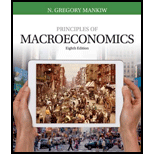# A market is described by the following supply and demand curves: Q S = 2 P Q D = 300 – P a. Solve for the equilibrium price and quantity. b. If the government imposes a price ceiling of $90, does a shortage or surplus (or neither) develop? What are the price, quantity supplied, quantity demanded, and size of the shortage or surplus? c. If the government imposes a price floor of$90, does a shortage or surplus (or neither) develop? What are the price, quantity supplied, quantity demanded, and size of the shortage or surplus? d. Instead of a price control, the government levies a tax on producers of $30. As a result, the new supply curve is: Q 5 = 2( P – 30). Does a shortage or surplus (or neither) develop? What are the price, quantity supplied, quantity demanded, and size of the shortage or surplus?BuyFindarrow_forward ### Principles of Macroeconomics (Mind... 8th Edition N. Gregory Mankiw Publisher: Cengage Learning ISBN: 9781305971509 #### Solutions Chapter SectionBuyFindarrow_forward ### Principles of Macroeconomics (Mind... 8th Edition N. Gregory Mankiw Publisher: Cengage Learning ISBN: 9781305971509 Chapter 6, Problem 10PA Textbook Problem 1325 views ## A market is described by the following supply and demand curves:QS = 2PQD = 300 – Pa. Solve for the equilibrium price and quantity.b. If the government imposes a price ceiling of$90, does a shortage or surplus (or neither) develop? What are the price, quantity supplied, quantity demanded, and size of the shortage or surplus?c. If the government imposes a price floor of $90, does a shortage or surplus (or neither) develop? What are the price, quantity supplied, quantity demanded, and size of the shortage or surplus?d. Instead of a price control, the government levies a tax on producers of$30. As a result, the new supply curve is:  Q5 = 2(P – 30).  Does a shortage or surplus (or neither) develop? What are the price, quantity supplied, quantity demanded, and size of the shortage or surplus?

Subpart (a):

To determine

Equilibrium price and equilibrium quantity.

### Explanation of Solution

Here, we have given the demand equation as well as the supply equation. We have to calculate the equilibrium price and quantity. The equilibrium price can be calculated by equating the demand equation with the supply equation in the economy as follows:

Demand=Supply300P=2P3P=300P=3003=100

Thus, the equilibrium price in the economy is \$100

Subpart (b):

To determine

Equilibrium price and equilibrium quantity with price ceiling.

Subpart (c):

To determine

Equilibrium price and equilibrium quantity with price floor.

Subpart (d):

To determine

Equilibrium price and equilibrium quantity with tax.

### Still sussing out bartleby?

Check out a sample textbook solution.

See a sample solution

#### The Solution to Your Study Problems

Bartleby provides explanations to thousands of textbook problems written by our experts, many with advanced degrees!

Get Started

Find more solutions based on key concepts
Why might economic advisers to the president disagree about a question of policy?

Brief Principles of Macroeconomics (MindTap Course List)

In what ways is economics a science?

Essentials of Economics (MindTap Course List)

Why is the NFV of a relatively long-term project (one for which a high percentage of its cash flows occurs in t...

Fundamentals of Financial Management, Concise Edition (with Thomson ONE - Business School Edition, 1 term (6 months) Printed Access Card) (MindTap Course List)

What is a relation? What is a tuple?

Pkg Acc Infor Systems MS VISIO CD

What is a nondiscretionary bonus?

PAYROLL ACCT.,2019 ED.(LL)-TEXT